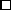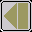### Theorem D

For all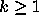,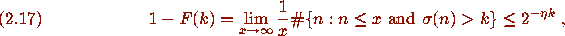where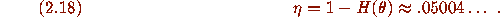Here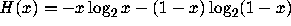is the entropy function and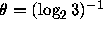.

### Proof D

Let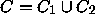, where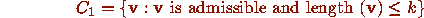and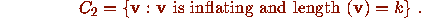Then C has the property that for any binary word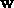of length k there is a unique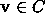with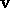a prefix of. Now for anywith length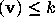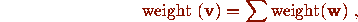where the sum is over allof length k for whichis a prefix of. Hence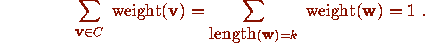From (2.16) this implies that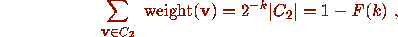where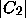denotes the number of vectors in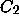. The already proved first part of Theorem A shows that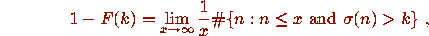so that to prove (2.17) it suffices to boundfrom above.

Now the definition (2.15) of an inflating vector implies that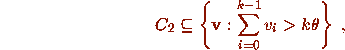so that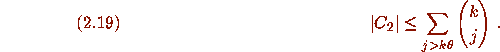The right side of (2.19) is just the tail of the binomial distribution. It is easily checked using Stirling's formula that for any constant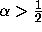and any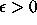the bound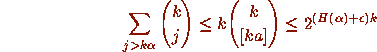holds for all sufficiently large k. With more work one can obtain the more precise estimate (Ash , Lemma 4.7.2) that for any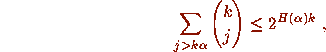which used in (2.19) implies (2.17).